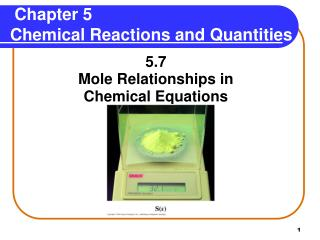# Chapter 5 Chemical Reactions and Quantities - PowerPoint PPT PresentationDownload PresentationChapter 5 Chemical Reactions and Quantities

Chapter 5 Chemical Reactions and QuantitiesDownload Presentation## Chapter 5 Chemical Reactions and Quantities

- - - - - - - - - - - - - - - - - - - - - - - - - - - E N D - - - - - - - - - - - - - - - - - - - - - - - - - - -
##### Presentation Transcript

1. Chapter 5 Chemical Reactions and Quantities 5.7 Mole Relationships in Chemical Equations

2. Law of Conservation of Mass The Law of Conservation of Massindicates that in an ordinary chemical reaction, • Matter cannot be created nor destroyed. • No change in total mass occurs in a reaction. • Mass of products is equal to mass of reactants.

3. Conservation of Mass 2 moles Ag + 1 moles S = 1 mole Ag2S 2 (107.9 g) + 1(32.1 g) = 1 (247.9 g) 247.9 g reactants = 247.9 g product

4. Reading Equations In Moles Consider the following equation: 4 Fe(s) + 3 O2(g) 2Fe2O3(s) This equation can be read in “moles” by placing the word “moles” between each coefficient and formula. 4 moles Fe + 3 moles O22 moles Fe2O3

5. Writing Mole-Mole Factors A mole-mole factor is a ratio of the moles for any two substances in an equation. 4Fe(s) + 3O2(g) 2Fe2O3(s) Fe and O24 moles Fe and 3 moles O2 3 moles O2 4 moles Fe Fe and Fe2O34 moles Fe and 2 moles Fe2O3 2 moles Fe2O3 4 moles Fe O2 and Fe2O33 moles O2 and 2 moles Fe2O3 2 moles Fe2O3 3 moles O2

6. Learning Check Consider the following equation: 3 H2(g) + N2(g) 2 NH3(g) A. A mole-mole factor for H2 and N2 is 1) 3 moles N22) 1 mole N2 3) 1 mole N2 1 mole H2 3 moles H2 2 moles H2 B. A mole-mole factor for NH3 and H2 is 1) 1 mole H22) 2 moles NH3 3) 3 moles N2 2 moles NH3 3 moles H2 2 moles NH3

7. Solution 3H2(g) + N2(g) 2NH3(g) A. A mole-mole factor for H2 and N2 is 2) 1 mole N2 3 moles H2 B. A mole-mole factor for NH3 and H2 is 2) 2 moles NH3 3 moles H2

8. Calculations with Mole Factors How many moles of Fe2O3 can form from 6.0 mole O2? 4Fe(s) + 3O2(g) 2Fe2O3(s) Relationship: 3 mole O2 = 2 mole Fe2O3 Write a mole-mole factor to determine the moles of Fe2O3. 6.0 mole O2 x 2 mole Fe2O3 = 4.0 mole Fe2O3 3 mole O2

9. Guide to Using Mole Factors

10. Learning Check How many moles of Fe are needed for the reaction of 12.0 moles O2? 4 Fe(s) + 3 O2(g) 2 Fe2O3(s) 1) 3.00 moles Fe 2) 9.00 moles Fe 3) 16.0 moles Fe

11. Solution 3) 16.0 moles Fe 12.0 moles O2 x 4 moles Fe = 16.0 moles Fe 3 moles O2